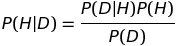# Posterior Probability

## What is the posterior probability?

In statistics, the posterior probability expresses how likely a hypothesis is given a particular set of data. In terms of conditional probability, we can represent it in the following way:

Posterior = P(H|D)

where D = data and H = hypothesis

This contrasts with the likelihood function, which is represented as P(D|H). This distinction is more of an interpretation rather than a mathematical property as both have the form of conditional probability. In order to calculate the posterior probability, we use Bayes theorem, which is discussed below.

Posterior and Bayes Theorem

Bayes theorem, which is the probability of a hypothesis given some prior observable data, relies on the use of likelihood P(D|H) alongside the prior P(H) and marginal likelihood P(D) in order to calculate the posterior P(H|D). The formula for Bayes theorem is:where D = data and H = hypothesis

Bayes theorem is a fundamental theorem in machine learning because of its ability to analyze hypotheses given some type of observable data. With this analysis, we can more accurately predict hypotheses on unseen data (note: see Black Swan Paradox). Since an agent is only able to view the world via the data it is given, it is important that it can extract reasonable hypotheses from that data and any prior knowledge. With the posterior, an agent is able to determine the validity of its hypothesis knowing the probability that expresses how likely the data is given its hypothesis and all prior observations.

## Example of Posterior Probability

Let’s say you’re at the grocery store and a person walking in front of you drops a \$5 bill. You pick it up and want to try to get their attention but you are unsure of whether to say “Excuse me Miss, or Sir”. All else being equal and based on the fact that there’s roughly equal populations of men and women on earth, the conditional probability that you say “Excuse me Miss” and it is in fact a woman that turns around is 0.5.

If we introduce new relevant information, say, that the person in question had long hair, we would have to update our conditional probability of the outcome that the person is in fact a woman. We would now have a new factor built into our probability calculation, giving us our posterior probability.

For a more detailed mathematical representation of the posterior probability and how to calculate it, see the Bayesian inference page.